The Electrodynamic Algorithms of Core of Stars and Black Holes | OMICS International
Journal of Physical Mathematics

# The Electrodynamic Algorithms of Core of Stars and Black Holes

Alexandris NG*

Independent Researcher, Greece

*Corresponding Author:
Nikos G Alexandris
Independent Researcher, Greece
Tel: 82991558855
E-mail: [email protected]

Received Date: May 05, 2016; Accepted Date: August 19, 2016; Published Date: August 22, 2016

Citation: Alexandris NG (2016) The Electrodynamic Algorithms of Core of Stars and Black Holes. J Phys Math 7: 192. doi: 10.4172/2090-0902.1000192

Copyright: © 2016 Alexandris NG. This is an open-access article distributed under the terms of the Creative Commons Attribution License, which permits unrestricted use, distribution, and reproduction in any medium, provided the original author and source are credited.

Visit for more related articles at Journal of Physical Mathematics

#### Abstract

The prediction of the magnetic field of Sgr A* by the algorithm of a star's core rotation is presented in author book “Modified Hawking field” 2010. In the present article author can see the way. Author can calculate the electrodynamic parameters of the nucleus of stars and black holes like Sgr A*.

#### Keywords

Gravity energy; Stoney's coefficient; Magnetic field; Black holes

#### Introduction

The following article is based on an idea of Alvfen Carlqvist  that author can predict the parameters of super massive stars and black holes by Electrodynamic parameters. Gravity energy is equal to electrodynamic energy and the magnetic field is produced by an L C circuit. The basic hypothesis of author book is that electric charge is equal to mass by a coefficient (k5.1a), different from Stoney's coefficient as well as Hawking's coefficient but it is in agreement with Hawking’s theory. In author mathematical method I introduce two mathematical parameters β and tc. These parameters are necessary to extract a coefficient which is related to the 2.73 K of cosmic background radiation .

Article

The prediction of magnetic field of Sgr A* by the algorithm of a star's core rotation is presented in author book . We can input to algorithm the mass of star m and the radius R=lc, lc=length of Coulomb law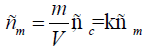(1)

ρm: density of mater, m: mass, V: volume=(4/3)π lc3, ρc: density of electric charge

k=k5.1a=(G/2πKe)1/2=3.43745 × 10-11 C/Kg,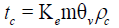(2)

Finally the tc parameter is independent of density of matter and it is depended on the radius (R3) of the star Ke: Coulomb constant, θV=(4/3) π, tc: mathematical parameter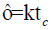(3)

τ: elastic coefficient of Hook law,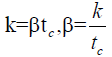(4)

We can find β mathematical parameter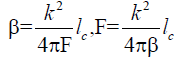(5)

lc is the radius(R) of the body, we find F force of oscilation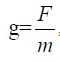, Newton law   (6)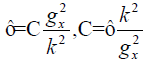(7)

we can find C capacity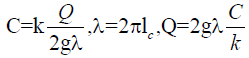(8)

We can find Q, the electric charge.

The period of rotation is: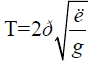(9)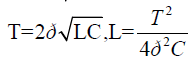(10)

L: self-induction coefficient.

So we can find the physics parameters of the star or black hole; g: acceleration, C: electric capacity, Q: electric charge, T: period of rotation and L: self-induction coefficient. From those values author can calculate the electromagnetic field of the core of a star or black hole energy of electric currents: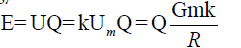(11)

U: electric potential, Um: gravitation potential , G: gravitation constant, m: mass of star, k=k5.1a

and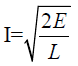(12)

I: intensity of current, L: self-induction coefficient so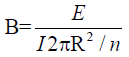(13)

Transformation of Lorenz-Laplace force F=B I 2πR/n, E=FR,

m: mass of star or black hole, Ι: intensity of current, R=radius of star, n=R/Rcore, Rcore: radius of core of star,

For black holes n=1, for Sun n=5 and for Earth n=2. The results are very good for Earth-nucleus, Sun and Sgr A*. Also author can write the function of magnetic field as following: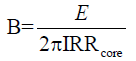(14)

For black holes R=Rcore

#### Magnetic Field of Sagittarius (Sgr A*) Black Hole

The event horizon experiment could prove Hawking-Bekenstein- Kerr theory . The mass of sagittarius black hole found, but we do not know the horizon surface and radius.

MBH=8.22 × 1036 kg

The radius of black hole arises from Hawking-Bekenstein-Kerr surface of horizon.

So RBH=1.1 × 1010m

Using the algorithm in my book "modified Hawking field" page 55- 56 we get the following values:

Q=4.4 × 1028 Cb C=190 F

I=4.9 × 1015 A B=0.02 Tesla

E=7.6 × 1034 Joule g=5.7 × 104 m/sec2

L=6200 H T=6.9 × 103 sec

V=2π R/T=1 × 107 m/sec, 3% of speed of light or 0.03 C.

This rotation function is appropriate for stars like the sun for this function rotation we use some of the six hypotheses. For a black hole it is better to use the following function:

Velocity of surface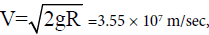11% the speed of light or 0.11 × C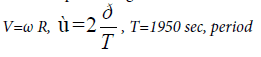All equations arise without relativity. Using the known mass of Sgr A* Black hole and the radius of Bekenstein–Hawking-Kerr function author can find the magnetic field of Black hole near the horizon without relativity[5-7]. The same algorithm is used for the Sun.

For the sun

The function is: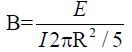(15)

Transformation of Lorenz-Laplace force

Ι=intensity of current, R=radius of Sun, R/5 is the radius of the core of the Sun, E=energy of the Sun's currents, B/10=surface magnetic field, 10 is the analogy of rotation between surface and core. The result for the core is 25 Gauss and for the surface 3.8 Gauss.

For the earth

Function (1) for Il2=IR2 gives 78 Gauss, for Il2=I(2ΠR)2 gives 2 Gauss, for Il2=I2ΠR2 gives 12 Gauss, for arises 24 Gauss which is the experimental value of 2010, R/2 is the radius of the Earth’s core. Also the algorithm gives rotation 1.3 days.

For neutron stars a few are in agreement with those functions like burst nebule.

Sgr A*

The algorithm gives 0.12 Tesla for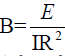, but the best function is: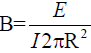, so the result is 0.02 Tesla, without relativity. I choose the relativistic coefficient,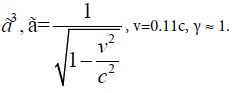I choose this coefficient for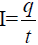and R2. For an observer far away from the horizon,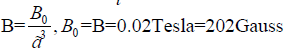(16)

This value 202 gauss is 500 times the Earth’s magnetic field . The algorithm uses coefficient k51a of all the above functions without strong participation of relativity. The problem of that algorithm is that does not give the rotation of black hole like the rotation of the Sun or the Earth. In 2013 88% the speed of light of the gas was observed

So the gases are falling and rotating under an acceleration g which is the same as the index. A good approximation could arise in the following way using relativity [4-8]: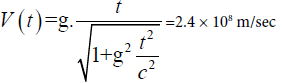(17)

or 0.8 C or 80% the speed of light, T=t If we use the constant of Stoney .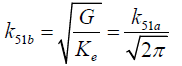(18)

We get for currents 0.3 C, B=200 Gauss and for rotation velocity 0.96 C. Stoney gives a great value.

#### The Paradox of the Hawking Theory

It is known that the Hawking-Bekenstein theory about black holes contains two basic equations. One of the equations is that of Bekenstein (1972) which correlates the surface of the horizon of a black hole to the mass of a black hole. Specifically, the surface is proportional to the square of the mass. The other equation is Hawking’s (1971) which connects the temperature of the horizon of a black hole with the mass. Temperature is inversely proportional to mass. These theories correlate fundamental theories of physics: the general relativity theory (not completely proven, due to our ignorance about gravity), quantum, thermodynamic and mechanical theory.

The paradox lies in the fact that no astronomical body gave the Hawking radiation or else the temperature which is monstrously 10-14 Kelvin small, weaker than the temperature of the universe, as Susskind protests in his recent book.

Sagittarius A*

Contemporary astrophysics has understood and photographed very dense objects, neutron stars. There has never been observed any body more dense than these, and the name black holes is attributed to them, without being able to confirm the Hawking-Bekenstein equations. The temperature (T) detected is much greater than 10-14 K. Kelvin and corresponds to X-ray and γ radiation. However, it is believed that it comes from clouds around the horizon rather than the horizon itself.

Μ?ζα Sagittarius A* (m): 1.2 × 1042 kg and for Black Hole ΒΗ 8.2 × 1036 kg    (19)

Radius (lc): 5 × 1014 m and for BH 1.1 × 1010m    (20)

Using the above values in the equation of page 48 which gives the temperatures of the cores of the stars, the result is temperature(T) similar to the one observed:

Radiation (T): 9.1 × 106 Kelvin or 6 Å and for BH 5.8 × 109 Kelvin or 0.5 Mev.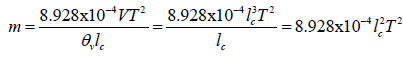(21)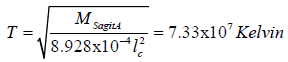and for BH 8.7 × 109kelvin=0.75 Mev

Colliders

This equation also gives the crushing length (lc) of the particles in the buffers. This is not odd because the crushing length (lc) corresponds to the wave formed on the particle which receives the energy of the collision (mass m). The particle becomes a crushing length sized star.

From (21) arises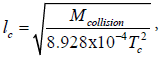(22)

For Mcollision=7 TeV/c2 and 1011 Kelvin arises lc=10-21 m

#### Hawking Theory

But what is the connection to the Hawking’s theory? If we correlate the above equation with the two Hawking-Bekenstein equations we might solve the paradox of the black hole temperature(T). On the equation of page 48 which gives the temperature(T) of the core of the stars we put the Planck temperature and the mass of Sagittarius A*(MsagitA). The result is the radius of an atom, which is very small.

From (22) arises: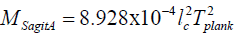then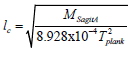(23)

lc=2.58 × 10-10 m, for BH lc=6.76 × 10-13m

If this length is imported as a horizon radius of the black hole facts we will take the mass(MB) of the black hole in the Bekenstein equation. This mass in the Hawking equation(MB=MH) will give the Hawking radiation(TH) which is about 12-20 times smaller than the observed.

Radius of Horizon of Black Hole of Bekenstein's12 function: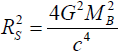(24)

Rs=lc, MB=1.74 × 1017 kg, for BH, MB=4.55 × 1014 kg

Radiation of Black Hole of Hawking's function: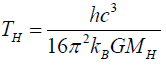(25)

MB=MH, TH=7 × 105 Kelvin, for BH TH=2.7 × 108 Kelvin=22.7 KeV

This value I in agreement with the observed values (2011 Fermi Symposium). This is the way that a paradox seems to be solved, but there is another one added. The black hole has a size of an atom. Mass MB must be singularity.

The theoretical paradox of the hawking theory

The general theory of relativity which is the gravity theory has given little evidence for 100 years, such as gravitational lenses galaxies. There has not, however, been proven that inertia is equivalent to gravity, or that acceleration of bodies produces gravitational waves.

For the same reason we do not yet accept that collisions of bodies and particles enhance gravity. In a few words we have not understood the nature of gravity. In the existing weakness of general relativity the Hawking theory introduces another assumption, that general relativity which applies in the macrocosm also applies in the region of quantum phenomena. Thus, either quantum effects apply in the macrocosm, or general relativity applies in the microcosm. The above calculations give an explanation. The great mass regards general relativity and small length of the black hole regards quantum. Therefore, perhaps the two theories become compatible. Although, we must not forget that paradoxes of black holes started from mechanics long before the appearance of relativity.

Paradox says that the escape velocity in a heavy body can reach the speed of light, so the star will be dark. In fact it will be so dark that it will also be completely cold. The latter has been a matter of controversy for the past 40 years. The problem is that it is not certain that a law of mechanics applies everywhere and in the same way. For example, logically it is not prohibited that the escape velocity is always less than the speed of light, so that the light escapes always.

Solar dynamo

The equations resulting from the assumptions can extract the rotation of stars and in particular of their cores, specifically the first 40 equations. Regarding the Sun’s rotation of the core it is 10 times faster than the surface, page 56. The fact that rotation concerns the core is shown by the fact that the same equations give the core’s temperature of sun 7 × 107 Kelvin, page 48. Confirmation of equations and of coefficients is very important for the rest part of the project. The coefficient of the equations selected so that they match with the empirical cosmological types. For the calculation of the magnetic field(B) the equation which gives the force is selected Laplace(F):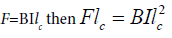(26)

So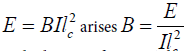(27)

The calculations of page 56 should be modified in order to take into account that the core of the sun has radius the 1/5 of the sun and that currents are not rectilinear but circular.

Eqn (27) arises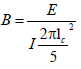(28) with I=2 × 107 Ampere

Energy (E) is proportional to the square of the angular speed, like the Laplace force:

Ε ≈ ω2. The product Ilc=Qv, (29)

speed (υ) is proportional to the angular speed (ω), thus the intensity of the magnetic field (B) is proportional to the angular speed ω.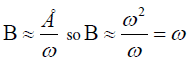(30)

Therefore, the magnetic field’s (B) intensity is inversely proportional to the rotation period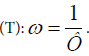. If the rotation of the surface is 10 times smaller, and therefore the magnetic field will be 10 times smaller, i.e. 44 Gaus/10=4.4 Gauss.

This verification shows that the coefficients of equations 1-47, pages 14-17 were selected properly. This verification enhances the accuracy of the temperature equation of the core of the star which is extracted from the same equations, pages 47-48. It is the equation that agrees with the radiance of Sagittarius A* and it is combined with the Hawking-Bekenstein equations. It also gives very good results to the collider regarding the length of particle breakage.

The special feature of the constant (CR) which gives the solar nuclear rotation of the star is that it depends on the constant k5.1a and not on the classic constant k5.1b of Stoney. Author should remind that the quotient of the electrical charge to the k5.1a gives Meg5.1a which is associated to the Planck mass and the Hawking and Stoney masses. The verification of the constant k5.1a is fundamental for the analysis of the book.

#### Conclusion

The algorithm can predict the basic parameters of a super massive star especially the nucleus and some planets like the Earth. The Stoney coefficient is appropriate for solid bodies where gravity and electricity are equal . The coefficient k5.1a is appropriate for states in nucleus matter of stars and some planets like the Earth. The bodies are different by an index n=R/Rcore which includes the analogy between the total radius of star and nucleus. The prediction is very good for magnetic field and rotation.

#### References

Select your language of interest to view the total content in your interested language

### Article Usage

• Total views: 8750
• [From(publication date):
September-2016 - Feb 22, 2020]
• Breakdown by view type
• HTML page views : 8629Can't read the image? click here to refresh